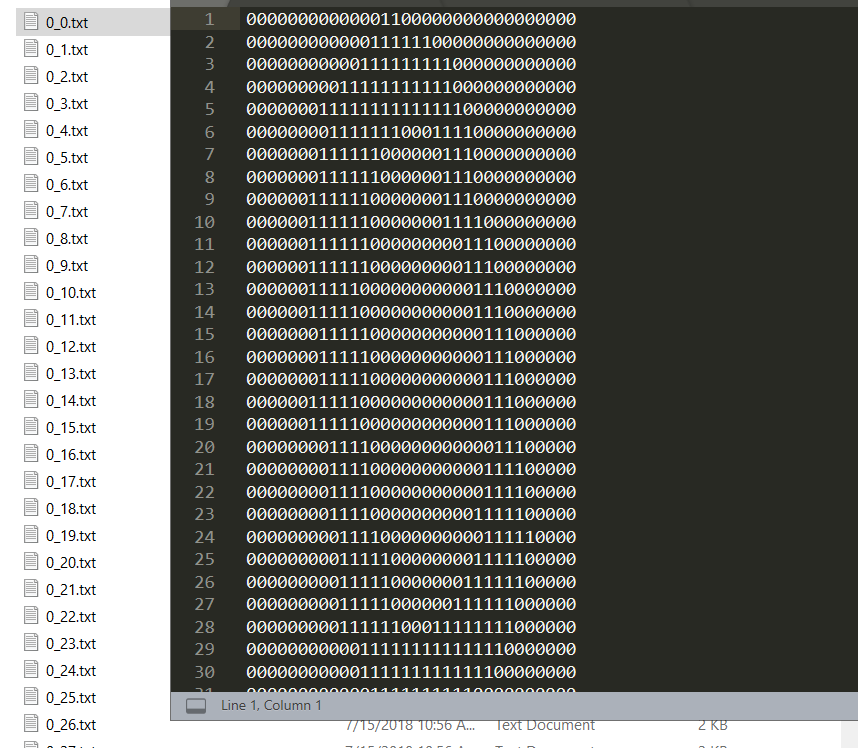## KNN (K近邻算法) - 识别手写数字

### KNN项目实战——手写数字识别

1、 介绍

k近邻法(k-nearest neighbor, k-NN)是1967年由Cover T和Hart P提出的一种基本分类与回归方法。它的工作原理是：存在一个样本数据集合，也称作为训练样本集，并且样本集中每个数据都存在标签，即我们知道样本集中每一个数据与所属分类的对应关系。输入没有标签的新数据后，将新的数据的每个特征与样本集中数据对应的特征进行比较，然后算法提取样本最相似数据(最近邻)的分类标签。一般来说，我们只选择样本数据集中前k个最相似的数据，这就是k-近邻算法中k的出处，通常k是不大于20的整数。最后，选择k个最相似数据中出现次数最多的分类，作为新数据的分类。

### 2、数据集介绍

32X32 文本格式数据.3、代码实现

3.1、导包

```import numpy as np
import pandas as pd
import matplotlib.pylab as plt
%matplotlib inline```
`import os`

3.2、读取训练数据

```# 获取数据文件
fileList = os.listdir('./data/trainingDigits/')

# 定义数据标签列表
trainingIndex = []
# 添加数据标签
for filename in fileList:
trainingIndex.append(int(filename.split('_')))

# 定义矩阵数据格式
trainingData = np.zeros((len(trainingIndex),1024))
trainingData.shape#(3868, 1024)```
```# 获取矩阵数据
index = 0
for filename in fileList:
with open('./data/trainingDigits/%s'%filename, 'rb') as f:

# 定义一个空矩阵
vect = np.zeros((1,1024))

# 循环32行
for i in range(32):
# 读取每一行数据

# 遍历每行数据索引  line[j] 即为数据
for j in range(32):
vect[0,32*i+j] = int(line[j])

trainingData[index,:] = vect
index+=1```

3.3、读取测试数据

```fileList2 = os.listdir('./data/testDigits/')

# 定义数据标签列表
testIndex = []
# 获取数据标签
for filename2 in fileList2:
testIndex.append(int(filename2.split('_')))

#读取测试数据

# 定义矩阵数据格式
testData = np.zeros((len(testIndex),1024))
testData.shape
#(946, 1024)

# 获取矩阵数据
index = 0
for filename2 in fileList2:
with open('./data/testDigits/%s'%filename2, 'rb') as f:

# 定义一个空矩阵
vect = np.zeros((1,1024))

# 循环32行
for i in range(32):
# 读取每一行数据

# 遍历每行数据索引  line[j] 即为数据
for j in range(32):
vect[0,32*i+j] = int(line[j])

testData[index,:] = vect
index+=1```

3.5、数据建模

```from sklearn.neighbors import KNeighborsClassifier

# 定义 k 为5个, 即 寻找最近的3个邻居
knn = KNeighborsClassifier(n_neighbors=3)

# 训练数据
knn.fit(trainingData,trainingIndex)```

3.6、分析数据

```%%time
# 预测数据

predict_data = knn.predict(testData)

# Wall time: 7.8 s

res = 0
for i in range(len(testIndex)):
if testIndex[i] == predict_data[i]:
res+=1

print("识别正确率: "+'%0.3f'%(res/len(testIndex)*100)+"%")
# 识别正确率: 98.626%```